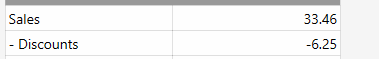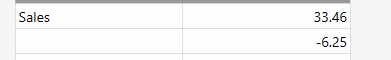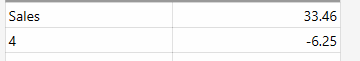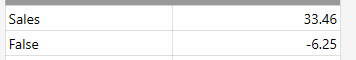# Use text in REPORT TICKET DETAILS

Is it possible to add plain text into report tag?

Currently I’m using this tag. This shows even when the total is 0.
`- Discounts|{REPORT TICKET DETAILS:=[CA.Discount.sum]+[CA.Round.sum]:(TY=Receipt) OR (TY=Refund)}`i want to try clean up the report and eliminate some of the figures that only occasionally come up.

Eg.
`{REPORT TICKET DETAILS:'-Discount',=[CA.Discount.sum]+[CA.Round.sum]:(TY=Receipt) OR (TY=Refund)}`

I often create a dummy field to get a blank space like: and the “space” does not print
`{REPORT TICKET DETAILS:space,=[CA.Discount.sum]+[CA.Round.sum]:(TY=Receipt) OR (TY=Refund)}`We can do simple math so hoping its possible to add some text.
`{REPORT TICKET DETAILS:="1+3",=[CA.Discount.sum]+[CA.Round.sum]:(TY=Receipt) OR (TY=Refund)}`Or
`{REPORT TICKET DETAILS:="1=3",=[CA.Discount.sum]+[CA.Round.sum]:(TY=Receipt) OR (TY=Refund)}`Try something like this (not tested):

``````{REPORT TICKET DETAILS:=[CA.Discount.sum]+[CA.Round.sum]:(TY=Receipt) OR (TY=Refund)::'Discount'|{0}}
``````
2 Likes

Perfect, Thanks. The `' '` on discount are not required.
`{REPORT TICKET DETAILS:=[CA.Discount.sum]+[CA.Round.sum]:(TY=Receipt) OR (TY=Refund)::Discount|{0}}`

1 Like